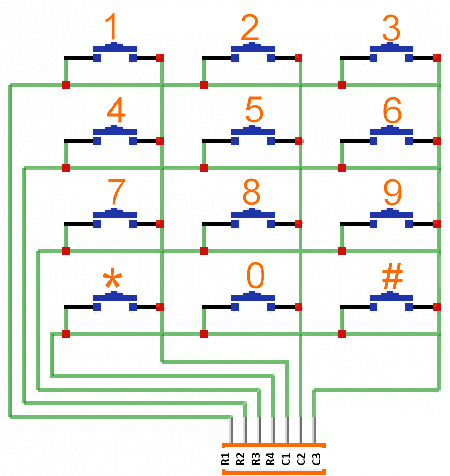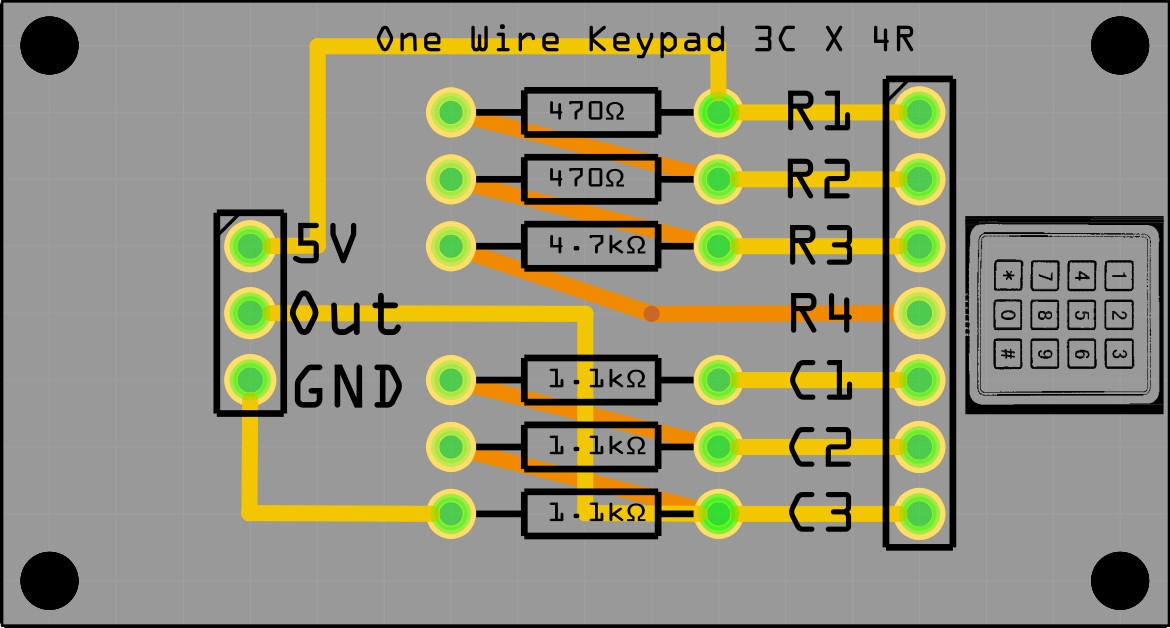This page describes how to wire the output of a seven pins, 3 columns * 4 rows key pad, to a one wire analogue output.
In this example only three resistor resistances are used.

There are many tutorials how to make a one-key wire keypad but or they use many resistors, make use of libraries or are hard to follow.

 In the picture on the right one can see that by pressing a button a connection is made between a row and a column. By choosing the right resistor combination the output will be different for every button. 5V is connected to C1 and ground after a 1.1 kilo-ohmresistor. When 3 is pressed the current flows from R1 tot C3 and flows to a 1.1 kilo-ohm resistor resulting in a reading of 989 bits. Pressing * R4 and C1 and connected resulting in a flow through all the resistors and a reading of 125 bits. To discriminate between the buttons the difference between the difference button must be as large as possible. Because resistors of identical resistance value will not have exact the same resistance measured, readings between modules will vary a little. In the Arduino source code the range per button is large enough to overcome these differences.Source code OneWireKeyPad.ino OneWireKeyPad.fzz Fritzing file Below the source code to read the key values
• ```int sensorPin = A0;   // select the input pin for the potentiometer
int ledPin = 13;      // select the pin for the LED
int sensorValue = 0;  // variable to store the value coming from the sensor
byte keyvalue;

void setup()
{
pinMode(ledPin, OUTPUT);   // declare the ledPin as an OUTPUT:
Serial.begin(9600);
Serial.println("Started. Press a key");
}

void loop()
{
digitalWrite(ledPin, HIGH);          // turn the ledPin on
switch(sensorValue)
{
case   0 ... 100:  keyvalue = 13; break;   // noise
case 101 ... 132:  keyvalue = 12; break;   // *
case 133 ... 154:  keyvalue =  0; break;   // 0
case 155 ... 216:  keyvalue = 11; break;   // #
case 217 ... 281:  keyvalue =  7; break;   // 7
case 282 ... 318:  keyvalue =  4; break;   // 4
case 319 ... 349:  keyvalue =  1; break;   // 1
case 350 ... 390:  keyvalue =  8; break;   // 8
case 391 ... 463:  keyvalue =  5; break;   // 5
case 464 ... 519:  keyvalue =  2; break;   // 2
case 520 ... 619:  keyvalue =  9; break;   // 9
case 620 ... 848:  keyvalue =  6; break;   // 6
case 849 ... 1023: keyvalue =  3; break;   // 3
}
if(keyvalue<13) { Serial.println(keyvalue); delay(300); }
digitalWrite(ledPin, LOW);   // turn the ledPin off:
}```
 Ed Nieuwenhuys, November 2019 < -- Back to start